# Tree Boosting With XGBoost – Why Does XGBoost Win “Every” Machine Learning Competition?

Synced tech analyst reviews the thesis "Tree Boosting With XGBoost - Why Does XGBoost Win 'Every' Machine Learning Competition", which investigates how XGBoost differs from traditional MART, and XGBoost's superiority in machine learning competition.

## Introduction

Tree boosting has empirically proven to be efficient for predictive mining for both classification and regression.

For many years, MART (multiple additive regression trees) has been the tree boosting method of choice. But a starting from 2015, a first to try, always winning algorithm surged to the surface: XGBoost. This algorithm re-implements the tree boosting and gained popularity by winning Kaggle and other data science competition.

In the thesis Tree Boosting With XGBoost – Why Does XGBoost Win “Every” Machine Learning Competition, the author Didrik Nielsen from Norwegian University of Science and Technology is trying to:

1. Investigate how XGBoost differs from traditional MART
2. Answer the question: why does XGBoost win “every” machine learning competition

The thesis is divided in three parts:

1. Review some core concepts of statistical learning
2. Introduce boosting and its interpretation as numerical optimization in function space; further more discuss tree methods and core elements of the tree boosting methods
3. Compare the properties of the tree boosting algorithms employed by MART and XGBoost; provide arguments for XGBoost’s popularity

## Basic Concepts of Statistical Learning

The paper introduce in first place the supervised learning task and discuss the model selection techniques.

The goal of a machine learning algorithm is to reduce the expected generalization error which is known as the risk. If we knew the true distribution P(x, y), risk minimization would be an optimization task solvable by an optimization algorithm. However, we do not know the true distribution but only a training set of samples. We need to convert it back into an optimization problem is to minimize the expected loss on the training set. Thus the empirical distribution defined by the training set replaces the true distribution. All above turns into the following statistical formula: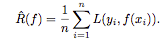Where $\hat{R}(f)$ is an empirical estimate of the true risk R(f) of a model. And L(.) refers to a loss function, such as squared error loss (common loss function for regression), other common loss functions can be found via here. n is the number of samples.

When n is large enough we have that：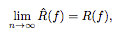ERM (Empirical Risk Minimization) is an induction principle which relies on minimization of the empirical risk (Vapnik, 1999). The empirical risk minimizer $\hat{f}$, is an empirical approximation of the target function, defined as:where F belongs to some class of functions, and referred as a model class, e.g. constant, linear methods, local regression methods (k-Nearest-Neighbors, kernel regression), splines, etc. ERM is a criterion to select the optimal function \hat{f} from a set of functions F.

The model class and ERM principle turns the learning problem into an optimization problem. The model class are considered candidate solutions function, while ERM principle provides us with a criterion to select the minimized function.

There are a vast set of methods for optimization problems, two prominent methods are gradient descent and Newton’s method which are used in MART and XGBoost respectively.

The author summarizes the common learning methods:

1. The constant
2. Linear methods
3. Local regression methods
4. Basis Function Expansions: explicit nonlinear terms, splines, kernel methods, etc…
5. Adaptive basis function models: GAMs (Generalized Additive Models), neural networks, tree models, boosting

Another machine learning concept is model selection, considering different learning methods and their hyper-parameters. The first question is always whether is it better to increase the complexity of a model And the answer always goes with the generalization performance of the model itself. As illustrated as the Fig. 1 below, we may have a better performance (avoid under-fitting) while the model is more complex, but we are losing the generalization performance (overfitting):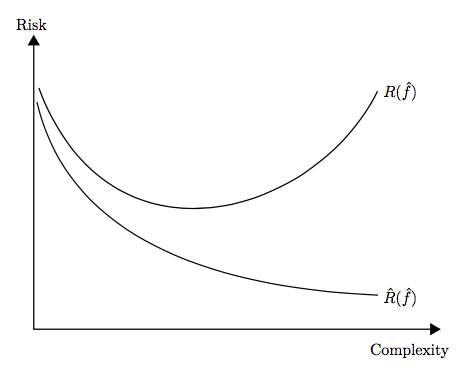Fig 1. Generalization vs training error

With the classic bias-variance decomposition of the expected conditional risk for the squared loss (only), we can visually observe the risk over complexity:Fig. 2 Expected Risk vs Variance vs Bias

One technique which is often used is regularization. By taking into account either implicitly or explicitly the fitness and imperfection of the data, regularization is the technique to control the variance of the fit. It also helps the models to be more generalized.

The complexity is measured differently across the model classes. LASSO (wikipedia::Lasso) and Ridge (Wikipedia::Tikhonov regularization) are two common measures for linear regression. We can apply the constraints (subsetting, step-wise) or the penalization (LASSO, Ridge) directly on the complexity measures.

## Understanding Boosting, Tree Methods & Tree Boosting

### Boosting

Boosting is a kind of learning algorithms that try to fit the data by using multiple simpler models, or so called base learner/weak learner. The way it does is to adaptively fit the data by using the same or slightly different in parameter setting’s base learners.

The very first development is AdaBoost by Freund and Schapire (1996). AdaBoost is actually minimizing the exponential loss function and iteratively get the weak classifier to be trained on weighted data. New boosting algorithm was also proposed which tries to minimize a second-order approximation of the log-loss: LogitBoost.

Breiman (1997a,b 1998) first developed the vision of boosting algorithms as numerical optimization techniques in function space. This vision enables the boosting technique can also be applied to regression problems. Two main numerical optimization approach were discussed in the thesis: gradient boosting and Newton boosting (also called second-order gradient boosting or Hessian boosting, as the Hessian Matrix is applied).

Let us develop the boosting algorithm step by step:

Boosting fits ensemble models of the kindWhich can be written as adaptive basis function modelswhere $f_{0}(x) = \theta_{0}$, $f_{m}(x) = \theta_{m}\phi_{m}(x) for m=1, …, M$, and $\phi_{m}$ is a sequentially add base functions to improve the fitness of the current model.

Thus most boosting algorithms can be seen to solveeither exactly or approximately at each iteration.

So, AdaBoost solves the above equation exactly for the exponential loss function under the constraint that $\phi_{m}$ are classifiers with A = {-1, 1}. And gradient boosting or Newton boosting solve the above equation approximately for any suitable loss function.

The algorithms of the gradient boosting and the newton boosting algorithm are given as follows: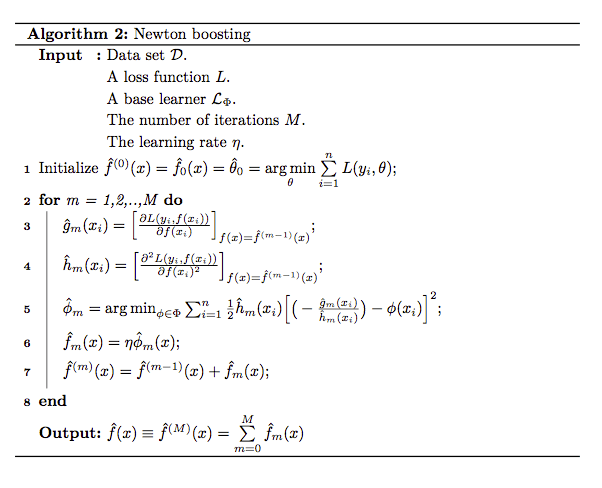• $\hat{g}_{m}(x_{i})$ is negative gradient
• $\hat{\phi}_{m}$ is the weak learner learnt with the data
• gradient boosting: $\hat{\rho}_m$ is the step length determined by line search
• Newton boosting: $\hat{h}_m$ is the empirical hessian

The most common used base learner is regression tree algorithm such as CART, and also component-wise linear models or component-wise smoothing splines. The principe of the base learner is to be simple, i.e. have high bias, but low variance.

The hyperparameters in the boosting methods are:

1. the number of iterations M: the bigger, higher chance of overfitting, thus a validation set or cross-validation required
2. the learning rate $\eta$: decreasing learning rate tends to improve generalization performance, but also increase M, as shown in the figure below.Out-of-sample RMSE for different learning rates on the Boston Housing dataset

### Tree Methods

Tree models are simple and interpretable models. They do have limited predictive power, but when multiple tree models are combined together as in bagged trees, Random Forest or in boosting, they become a powerful predictive model.

We can view tree models as partitioning the feature space into different set of rectangular and non-overlapping regions, then it fits some simple model in it. A visualization is shown below by using the Boston Housing data:The number of terminal nodes and the depth of the tree can be seen as the complexity measurement of tree models. In order to generalize the model, we can easily apply either a complexity constraints on the complexity measurement or a penalization on the number of terminal nodes or penalization of the leaf weights (used by XGBoost).

As learning the structure of the tree is NP-complete, the learning algorithms tend to compute an approximate solution. There are many different learning algorithms, such as CART (Classification And Regression Trees), C4.5 and CHAID. In the thesis, the author describes the CART because MART uses CART, XGBoost also implements a Tree model related to CART.

CART grows the tree in a top-down fashion. By considering every split parallel to the coordinate axes, CART chooses the split that minimizes the objective. In the second step, CART consider every split parallel within each regions. At the end of the iteration, the best split is chosen. And CART will repeat all the steps till the stopping criterion.

Given a region R_j, learning the weight w_j is typically straightforward. Let I_j denote the set of indices that belongs to region R_j, i.e. x_i ∈ R_j for i ∈ I_j.

The weight is estimated byFor a tree model \hat{f}, the empirical risk is given bywhere \hat{L}_{j} denote the aggregated loss at node j.

In the learning process, the current tree model is denoted by \hat{f}_{before} and \hat{f}_{after}\.

We can calculate the gain of the considered split by:For each split made, the gain is calculated for every possible split at every possible node and the split with maximal gain is taken.

Now let’s take a look at the missing values. CART handles the missing values by using surrogate variables, i.e. for each predictor, we only use non-missing data for finding the split, then based on primary split, we find a surrogate predictors to mimic the split. For example, suppose that, in a given model, CART splits data according to household income. If a value for income is not available, CART might substitute education level as a good surrogate.

However in XGBoost, the algorithm treats missing values by learning default directions. Internally, XGBoost will automatically learn what is the best direction to go when a value is missing. Equivalently, this can be viewed as automatically “learn” what is the best imputation value for missing values based on reduction on training loss.

Considering the categorical predictors, we can treat them in two different ways: grouped categories or independent categories. CART handles grouped categories, while the XGBoost requires independent categories (one-hot encoding).

Author summarize a list of pros and cons of the tree models:

Pros (Hastie et al., 2009; Murphy, 2012):

• are easily interpretable.
• are relatively fast to construct.
• can naturally deal with both continuous and categorical data.
• can naturally deal with missing data.
• are robust to outliers in the inputs.
• are invariant under monotone transformations of the inputs.
• perform implicit variable selection.
• can capture non-linear relationships in the data.
• can capture high-order interactions between inputs.
• scale well to large data sets.

Cons (Hastie et al., 2009; Kuhn and Johnson, 2013; Wei-Yin Loh, 1997; Strobl et al., 2006):

• tend to select predictors with a higher number of distinct values.
• can overfit when faced with predictors with many categories.
• are unstable and have high variance.
• lack smoothness.
• have difficulty capturing additive structure.
• tend to have limited predictive performance.

### Tree Boosting

With all the above development, now we come to combine the boosting algorithm with the base learner tree methods.

The boosted tree models can be viewed as adaptive basis function models, where the basis functions are regression trees: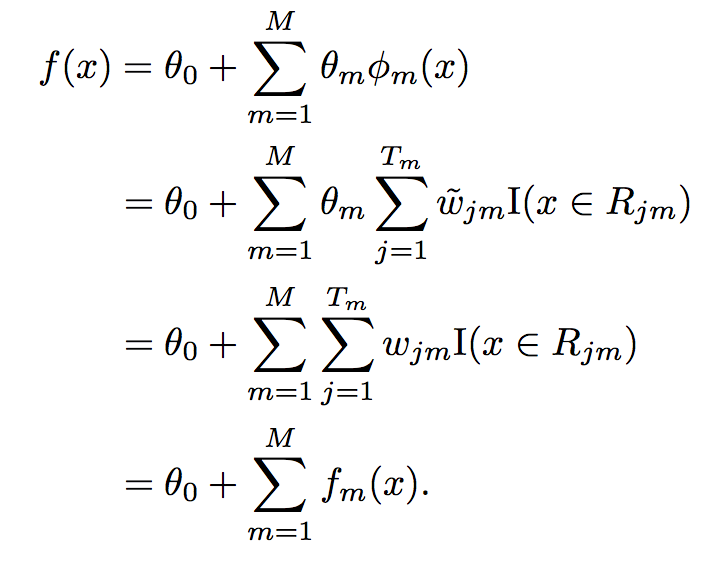Boosting tree model is a sum of multiple trees $f_{m}$, so it’s also called tree ensembles or additive tree models. Hence it’s smoother than a single tree model as seen in the figure below:Visualization of an additive tree model fit to the Boston Housing data

There are many ways to achieve the regularization on boosted tree models:

1. Regularization on the basis function expansion
2. Regularization on the individual tree model
3. Randomization

In general, the boosted tree tend to use shallow regression trees, i.e. regression trees with few terminal nodes, which has lower variance, but higher bias compared to deeper trees. This can be done by applying applying complexity contraints.

One of the improvement of XGBoost over MART is the penalization of complexity, which was not common for additive tree models. The penalization terms of the objective function can be written as:which the first term is the number of terminal nodes of each individual tree, the second is the L2 regularization on the term weights, the last one is the L1 regularization on the term weights.

The randomization was first introduced by Friedman(2002) through stochastic gradient descent which include row subsampling at each iteration. The randomization help to improve the generalization performance. There are two subsampling ways: row subsampling, column subsampling. MART includes only row subsampling (without replacement), while XGBoost includes both row and column subsampling.

As already discussed before, MART and XGBoost use two different boosting algorithms for fitting additive tree models. They are named GTB (gradient tree boosting) and NTB (Newton tree boosting) respectively. Both algorithms seek to minimize:at each iteration m, and the basis functions are trees:The general steps includes 3 stages:

1. Determine the leaf weights $\texttildelow{\omega}_{jm}$ for a fixed candidate tree structure.
2. Different tree structures are proposed with weights determined from the previous stage, therefore, the tree structure and and the regions are determined.
3. Once a tree structure is settled upon, the final leaf weights $\texttildelow{\omega}_{jm}, j=1,..,T$ in each terminal node are determined.

The algorithm 3 and 4 extends the 1 and 2 with trees as basis function:## Difference of XGBoost and MART

Finally, the author compares the details between two tree boosting algorithms and try to find out why XGBoost is better.

### Algorithms level comparison

As discussed in the previous sections, XGBoost and MART all try to solve the same empirical risk minimization problem: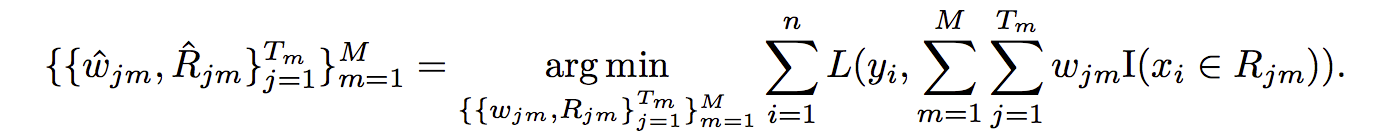by simplified FSAM (Forward Stage Additive Modelling), i.e. instead of greedy search, adding one tree at a time. At iteration m, a new tree is learnt using:XGBoost employs the algorithm 3 (above), the Newton tree boosting to approximate the optimization problem. And MART employs the algorithm 4 (above), the gradient tree boosting to do so. These two methods differs at first how they learn tree structures and then how they learn the leaf wights to assign in the terminal nodes of the learnt tree structure.

Revisiting the algorithms, we can find that Newton tree boosting has Hessian matrix which plays a crucial role to determine the tree structure, XGBoost: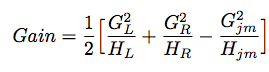while with gradient tree boosting, MART: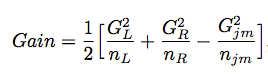Then, the leaf weights of Newton tree boosting, XGBoost is directly determined by: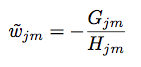while with gradient tree boosting, MART:In conclusion, XGBoost by using Hessian, a higher-order approximation, it learns a better tree structure. However, MART is better on determine the leaf weights, but for a less accurate tree structure.

In term of loss function applicability, Newton boosting requires the loss function to be twice differentiable as employing Hessian Matrix. So it is more strict on selecting loss function to be convex.

Once Hessian equals 1 everywhere, the two methods are equivalent, this is the case for squared error loss function. Thus, if we use any loss function other than squared error loss, Newton tree boosting, XGBoost should do better on tree structure learning. Instead gradient tree boosting is more accurate on leaf weights subsequently. Thus mathematically, they are not comparable.

Nevertheless, the author test them on two standard datasets: the sonar and the Ionosphere (Lichman, 2013). The empirical comparison was done on trees with 2 terminal nodes and no other regularization, and the data having no categorical features nor missing values. A line search is also added for gradient tree boosting shown in red.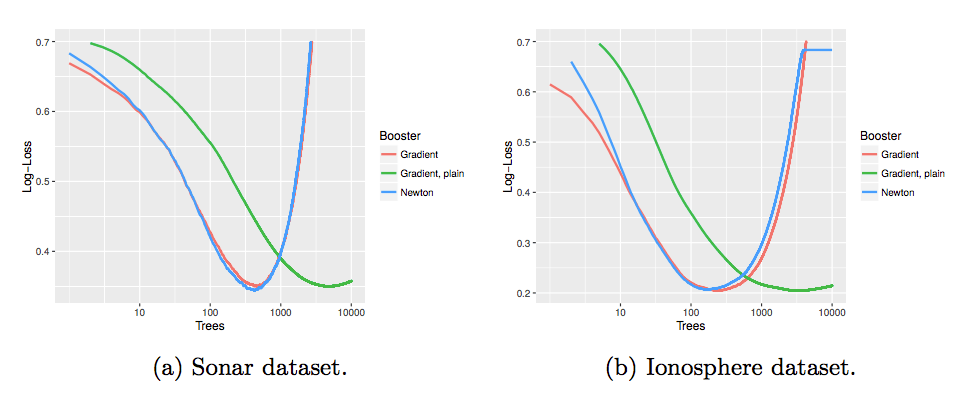The comparison chart shows that the two methods performs seamlessly. And the line search really improves the rate of convergence for gradient boosting tree.

### Regularization comparison

There are essentially three types of regularization parameter:

1. boosting parameters: number of trees M and the learning rate $\eta$
2. tree parameters: constraints and penalties on the complexities of individual trees
3. randomization parameters: row and column subsampling

The main difference of two boosting methods concentrate on tree parameters, as well as randomization parameters.

For tree parameters, in MART, each tree has the same number of terminal nodes, but for XGBoost, the terminal node penalization $\gamma$ might be included, thus the number of terminal nodes might vary and bounded by a maximum number of terminal nodes. XGBoost also implement the l2 regularization on leaf weights, and will implement L1 regularization on leaf weights.

On randomization parameters, XGBoost provides column subsampling in addition to row subsampling which is the only randomization parameter provided by MART.

### Why does XGBoost win “every” competition?

By using simulated data, authors firstly shows that tree boosting can be seen to adaptively determine the local neighbourhoods.

Usingto generateand fit it by local linear regression with two different degrees of flexibility fit: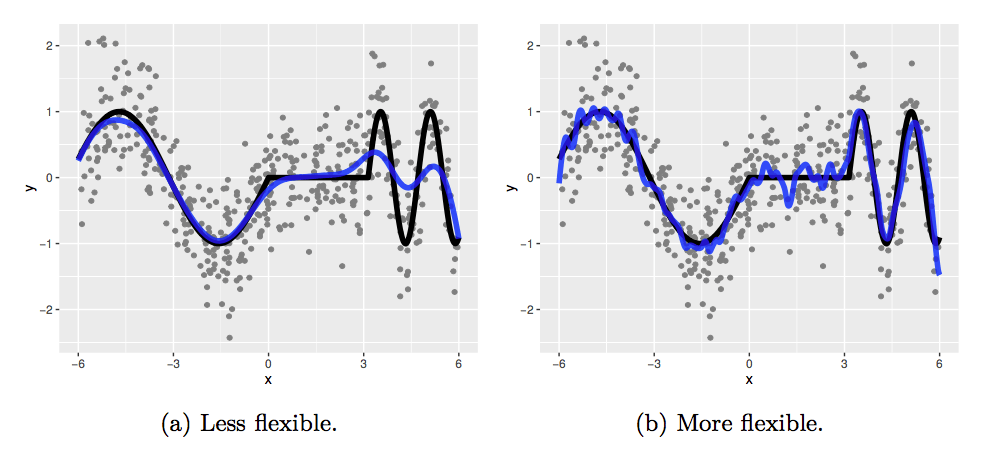and fit it by smoothing splines with two different degrees of flexibility fit:Now we try boosted tree stumps (two terminal nodes) fit: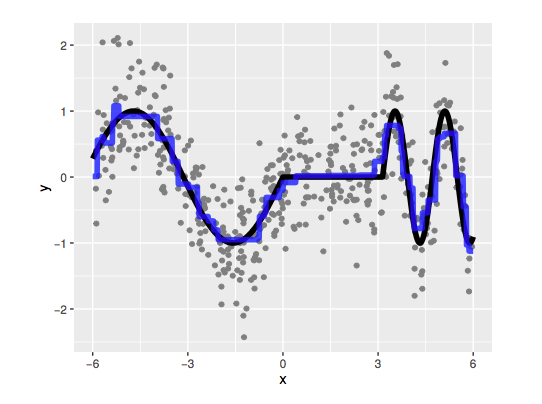Author details how weight function affects the determination of the fit and shows that the tree boosting can be seen to directly take the bias-variance tradeoff into consideration during fitting. This helps the neighbourhoods are kept as large as possible in order to avoid increasing variance unnecessarily, and only made smaller when complex structure seems apparent.

While coming to high dimensional problem, Tree boosting “beats” the curse of dimensionality by not relying on any distance metric. Also the similarity between data points are learnt from the data through adaptive adjustment of neighbourhoods. This makes the model immune to the curse of dimensionality.

Also deeper trees help to capture the interaction of the features. Thus there will be no need to search for appropriate transformations.

Thus, with the benefits of boosting tree models, i.e. adaptively determined neighbourhoods, MART and XGBoost in general should make a better fit than other methods. They are able to perform automatic feature selection and capture high-order interactions without breaking down.

By comparing MART and XGBoost, while MART does only set equal number of terminal nodes across all trees, XGBoost set a $T_{max}$ and regularization parameter to make the tree deeper while still keeping the variance lower. The Newton boosting used by XGBoost is likely to learn better structures compared to MART’s gradient boosting. XGBoost also includes an extra randomization parameter, i.e. column subsampling, this help to reduce the correlation of each tree even further.

## Reviewer’s thoughts

The author starts from the basics to the detail. The thesis help readers to understand the behind scene algorithm of boosting tree methods. By doing empirical and simulated comparisons, we can better grasp the key advantage of boosting tree compared to other models and comprehend how XGBoost outperforms in general MART. Hence, we could state that XGBoost brings new ways to improve the boosting tree.

The reviewer has participated in several Kaggle competitions. He has notified public interest of XGBoost and vast and in-depth discussion of how to tune the XGBoost’s hyper-parameters. He believes this article enlightens and help the beginner and intermediate competitor to better understand XGBoost in details.

## Reference

Chen, T. and Guestrin, C. (2016). Xgboost: A scalable tree boosting system. In Proceedings of the 22Nd ACM SIGKDD International Conference on Knowl- edge Discovery and Data Mining, KDD ’16, pages 785–794, New York, NY, USA. ACM.

Freund, Y. and Schapire, R. E. (1996). Experiments with a new boosting algorithm. In Saitta, L., editor, Proceedings of the Thirteenth International Conference on Machine Learning (ICML 1996), pages 148–156. Morgan Kaufmann.

Friedman, J. H. (2002). Stochastic gradient boosting. Comput. Stat. Data Anal., 38(4):367–378.

Hastie, T., Tibshirani, R., and Friedman, J. (2009). The Elements of Statistical Learning: Data Mining, Inference, and Prediction, Second Edition. Springer Series in Statistics. Springer.

Kuhn, M. and Johnson, K. (2013). Applied Predictive Modeling. SpringerLink : Bu ̈cher. Springer New York.

Lichman, M. (2013). UCI machine learning repository.

Murphy, K. P. (2012). Machine Learning: A Probabilistic Perspective. The MIT Press.

Strobl, C., laure Boulesteix, A., and Augustin, T. (2006). Unbiased split selection for classification trees based on the gini index. Technical report.

Vapnik, V. N. (1999). An overview of statistical learning theory. Trans. Neur. Netw., 10(5):988–999.

Wei-Yin Loh, Y.-S. S. (1997). Split selection methods for classification trees. Statistica Sinica, 7(4):815–840.

Wikipedia::Lasso. https://en.wikipedia.org/wiki/Lasso_(statistics)

Wikipedia::Tikhonov regularization. https://en.wikipedia.org/wiki/Tikhonov_regularization

Technical Analyst:  Yi Jin | Localization: Joni Chuang

Source: https://brage.bibsys.no/xmlui/bitstream/handle/11250/2433761/16128_FULLTEXT.pdf?sequence=1&isAllowed=y### About Synced

Machine Intelligence | Technology & Industry | Information & Analysis